JMSLTM Numerical Library 7.2.0
com.imsl.stat

## Class KolmogorovTwoSample

• All Implemented Interfaces:
Serializable

```public class KolmogorovTwoSample
extends Object
implements Serializable```
Performs a Kolmogorov-Smirnov two-sample test.

Class `KolmogorovTwoSample` computes Kolmogorov-Smirnov two-sample test statistics for testing that two continuous cumulative distribution functions (CDF's) are identical based upon two random samples. One- or two-sided alternatives are allowed. Exact p-values are computed for the two-sided test when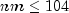, where n is the number of non-missing X observations and m the number of non-missing Y observation.

Let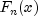denote the empirical CDF in the X sample, let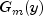denote the empirical CDF in the Y sample and let the corresponding population distribution functions be denoted by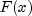and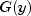, respectively. Then, the hypotheses tested by `KolmogorovTwoSample` are as follows: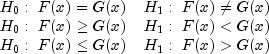The test statistics are given as follows: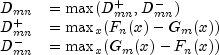Asymptotically, the distribution of the statistic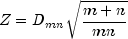converges to a distribution given by Smirnov (1939).

Exact probabilities for the two-sided test are computed when, according to an algorithm given by Kim and Jennrich (1973). When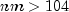, the very good approximations given by Kim and Jennrich are used to obtain the two-sided p-values. The one-sided probability is taken as one half the two-sided probability. This is a very good approximation when the p-value is small (say, less than 0.10) and not very good for large p-values.

Example, Serialized Form
• ### Constructor Summary

Constructors
Constructor and Description
```KolmogorovTwoSample(double[] x, double[] y)```
Constructs a two sample Kolmogorov-Smirnov goodness-of-fit test.
• ### Method Summary

Methods
Modifier and Type Method and Description
`double` `getMaximumDifference()`
Returns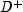, the maximum difference between the theoretical and empirical CDF's.
`double` `getMinimumDifference()`
Returns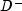, the minimum difference between the theoretical and empirical CDF's.
`int` `getNumberMissingX()`
Returns the number of missing values in the `x` sample.
`int` `getNumberMissingY()`
Returns the number of missing values in the `y` sample.
`double` `getOneSidedPValue()`
Probability of the statistic exceeding D under the null hypothesis of equality and against the one-sided alternative.
`double` `getTestStatistic()`
Returns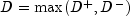.
`double` `getTwoSidedPValue()`
Probability of the statistic exceeding D under the null hypothesis of equality and against the two-sided alternative.
`double` `getZ()`
Returns the normalized D statistic without the continuity correction applied.
• ### Methods inherited from class java.lang.Object

`clone, equals, finalize, getClass, hashCode, notify, notifyAll, toString, wait, wait, wait`
• ### Constructor Detail

• #### KolmogorovTwoSample

```public KolmogorovTwoSample(double[] x,
double[] y)```
Constructs a two sample Kolmogorov-Smirnov goodness-of-fit test.
Parameters:
`x` - is an array containing the observations from the first sample.
`y` - is an array containing the observations from the second sample.
• ### Method Detail

• #### getMaximumDifference

`public double getMaximumDifference()`
Returns, the maximum difference between the theoretical and empirical CDF's.
Returns:
The value.
• #### getMinimumDifference

`public double getMinimumDifference()`
Returns, the minimum difference between the theoretical and empirical CDF's.
Returns:
The value.
• #### getNumberMissingX

`public int getNumberMissingX()`
Returns the number of missing values in the `x` sample.
Returns:
The number of missing values in `x`.
• #### getNumberMissingY

`public int getNumberMissingY()`
Returns the number of missing values in the `y` sample.
Returns:
The number of missing values in `y`.
• #### getOneSidedPValue

`public double getOneSidedPValue()`
Probability of the statistic exceeding D under the null hypothesis of equality and against the one-sided alternative. An exact probability is computed if the number of observation is less than or equal to 80, otherwise an approximate probability is computed.
Returns:
the one-sided probability.
• #### getTestStatistic

`public double getTestStatistic()`
Returns.
Returns:
The value D.
• #### getTwoSidedPValue

`public double getTwoSidedPValue()`
Probability of the statistic exceeding D under the null hypothesis of equality and against the two-sided alternative. This probability is twice the probability,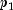, reported by `getOneSidedPValue`, (or 1.0 if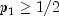. This approximation is nearly exact when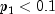.
Returns:
the two-sided probability.
• #### getZ

`public double getZ()`
Returns the normalized D statistic without the continuity correction applied.
Returns:
the value Z
JMSLTM Numerical Library 7.2.0